# 9. Classification of equilibrium points in specific systems.

Again consider the systemThe equilibrium point obviously is (0,0). Letandbe the eigenvalues to the matrix A. We have the following different possibilities:

1) IfLet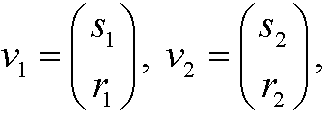be the eigenvectors belonging to the corresponding eigenvaluesand. Then the general solution of the system above isWe again have a number of possibilities:
i)   c2=0,
c1>0x(t) is a curve along l1+ (away from origo for increasing t).
ii)
c2=0, c1<0x(t) is a curve along l1 (away from origo for increasing t).
iii) c1=0, c2>0x(t) is a curve along l2+ (away from origo for increasing t).
iv) c1=0, c2<0x(t) is a curve along l2 (away from origo for increasing t).v) neither c1 nor c2 are zero. Then forwe havehencefor large (in absolute value) negative t. In particular we have that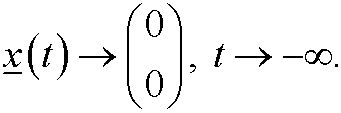When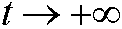we have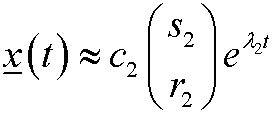for large positive t. Hence x(t) diverges to infinity with a slope asympotically v2 as t goes to (positive) infinity. This means that (0,0) is an unstable node. The lines defined by the eigenvectors v1 and v2 are called separatrices. The behavior is shown below in the phase portrait.2) IfIn the same way as above we realize that (0,0) is a stable node. The phase portrait looks the same but with reversed arrows.

3) IfWe have the following possibilities:
i)   c2=0, c1>0x(t) is a curve along l1+ (towards origo for increasing t).
ii)
c2=0, c1<0x(t) is a curve along l1 (towards origo for increasing t).
iii) c1=0, c2>0x(t) is a curve along l2+ (away from origo for increasing t).
iv) c1=0, c2<0x(t) is a curve along l2 (away from origo for increasing t).

We have a saddle point. See the phase portrait below.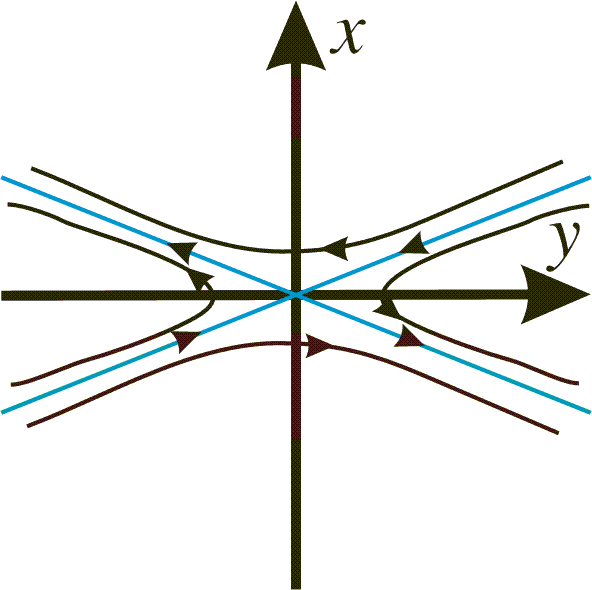4) If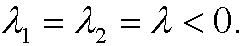We have two cases:

a) We have two linearly independent eigenvectorsThen we can write the solutionwhere a1 and a2 are arbitrary. This means that every curve is a halfline towards origo. See the phase portrait below.b) We only have one eigenvector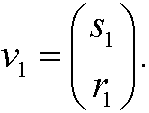Then the solution on the form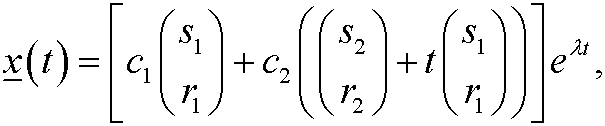where the vectorsatisfies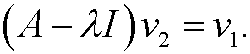For large t this means that the solution is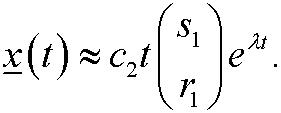In this case the phase portrait will look like this.Both these cases are examples of a stable node.

5) IfThis case is analogous to case 4) above, but with reversed arrows; (0,0) is an unstable node.

6) IfBy using similar arguments as in Example 9 we realize that the real solutions areThree cases:
i): Then x(t) and y(t) are periodic with period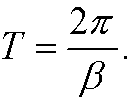The point (0,0) is a center.

ii)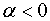: The amplitude of x decreases and we thus have a stable spiral.

iii)The amplitude of x increases and we thus have an unstable spiral.Example 10:
Consider the systemThe coefficient matrixhas the characteristic equationand thus the eigenvaluesThis corresponds to case 3) above and we conclude that (0,0) is an unstable saddle point. The eigenvectors are obtained by solving the linear equation system: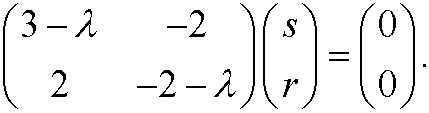Eigenvalue 1:implies thatthat is, the corresponding eigenvector is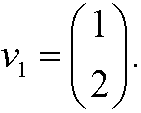Eigenvalue 2: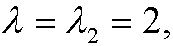implies thatthat is, the corresponding eigenvector is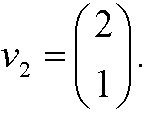The general solution of the system thus is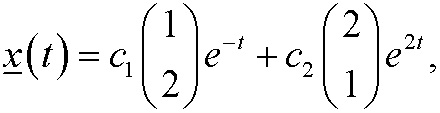that isThe eigenvectors define the directions of the separatrices.Example 11:
Consider the systemHere we have the critical point (2,1). We make the change of variables x1=x-2 and y1=y-1 and rewrite the system as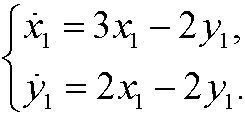By using the result from example 10 above we see that the point (2,1) is an unstable saddle point and that the solution to the original system isWe get the phase portrait by taking that from example 10 and move it two steps in the x-direction and one step in the y-direction.
In the same way as in Example 11 we may instead study the more general systemThe equlibrium point is here (a0,b0). By making the change of variables x1=x-a0 and y1=y-b0 we can transfer the system to the ones studied above with equilibrium point (0,0).